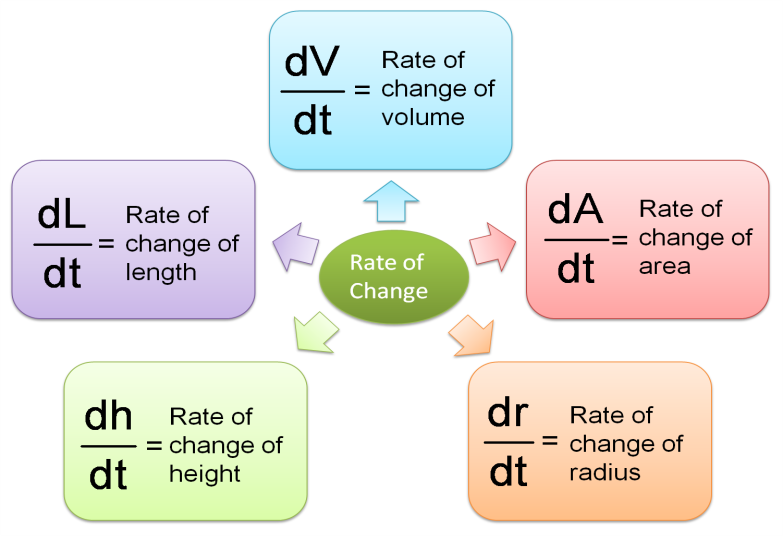# 9.8 Related Rates of Change

(A) Related Rates of Change

1. If two variables and y are connected by the equation y = f(x)

Notes:
If x changes at the rate of 5 cms -1   $\frac{dx}{dt}=5$
Decreases/leaks/reduces Þ  NEGATIVES values!!!

Example 1 (Rate of change of y and x)
Two variables, and y are related by the equation   $y=4x+\frac{3}{x}$ . Given that y increases at a constant rate of 2 units per second, find the rate of change of x when x = 3.

Solution:

(B) Rates of Change of Volume, Area, Radius, Height and Length(C) Rate of Change of Any Combination of Two Variables